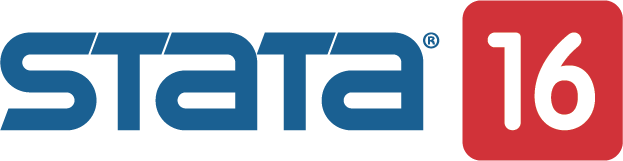# Learn Stata

### Univariate Time Series with Stata## NetCourse®461: Univariate Time Series with Stata

Course length: 6 weeks (4 lessons)

Dates: 3 April – 22 May 2020

See future dates.

## Enrol:

#### Content:

Learn about univariate time-series analysis with an emphasis on the practical aspects most needed by practitioners and applied researchers. Written for a broad array of users, including economists, forecasters, financial analysts, managers, and anyone who wants to analyze time-series data. Become expert in handling date and date–time data, time-series operators, time-series graphics, basic forecasting methods, ARIMA, ARMAX, and seasonal models.

We provide lesson material, detailed answers to the questions posted at the end of each lesson, and access to a discussion board on which you can post questions for other students and the course leader to answer.

#### Prerequisites:

• Stata 16 installed and working
• Course content of NetCourse 101 or equivalent knowledge
• Familiarity with basic cross-sectional summary statistics and linear regression
• Internet web browser, installed and working

Note: This course is platform independent. You can use Stata on Windows, Mac or Linux.

#### Trainer: Miguel Dorta#### Trainer: Gustavo Sánchez#### Course content

Lesson 1: Introduction

• Course outline
• What is so special about time-series analysis?
• Time-series data in Stata
• The basics
• Clocktime data
• Time-series operators
• The lag operator
• The difference operator
• The seasonal difference operator
• Combining time-series operators
• Working with time-series operators
• Parentheses in time-series expressions
• Percentage changes
• Drawing graphs
• Basic smoothing and forecasting techniques
• Four components of a time series
• Moving averages
• Exponential smoothing
• Holt–Winters forecasting

Lesson 2: Descriptive analysis of time series

• The nature of time series
• Stationarity
• Autoregressive and moving-average processes
• Moving-average processes
• Autoregressive processes
• Stationarity of AR processes
• Invertibility of MA processes
• Mixed autoregressive moving-average processes
• The sample autocorrelation and partial autocorrelation functions
• A detour
• The sample autocorrelation function
• The sample partial autocorrelation function
• A brief introduction to spectral analysis—The periodogram

Lesson 3: Forecasting II: ARIMA and ARMAX models

• Basic ideas
• Forecasting
• Two goodness-of-fit criteria
• More on choosing the number of AR and MA terms
• Seasonal ARIMA models
• Multiplicative seasonality
• ARMAX models
• Intervention analysis and outliers
• Final remarks on ARIMA models

Note: There is a one-week break between the posting of Lessons 3 and 4; however, course leaders are available for discussion.

Lesson 4: Regression analysis of time-series data

• Basic regression analysis
• Autocorrelation
• The Durbin–Watson test
• Other tests for autocorrelation
• Estimation with autocorrelated errors
• The Newey–West covariance matrix estimator
• ARMAX estimation
• Cochrane–Orcutt and Prais–Winsten methods
• Lagged dependent variables as regressors
• Dummy variables and additive seasonal effects
• Nonstationary series and OLS regression
• Unit-root processes
• ARCH
• A simple ARCH model
• Testing for ARCH
• GARCH models
• Extensions

Note: The previous four lessons constitute the core material of the course. The following lesson is optional and introduces Stata’s multivariate time-series capabilities.

Bonus lesson: Overview of multivariate time-series analysis using Stata

• VARs
• The VAR(p) model
• Lag-order selection
• Diagnostics
• Granger causality
• Forecasting
• Impulse–response functions
• Orthogonalized IRFs
• VARX models
• VECMs
• A basic VECM
• Fitting a VECM in Stata
• Impulse–response analysis